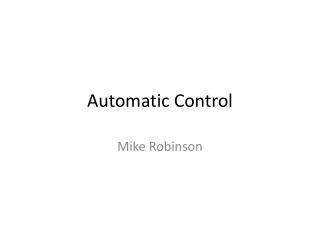DownloadDownload PresentationAutomatic Control

# Automatic Control

Download Presentation## Automatic Control

- - - - - - - - - - - - - - - - - - - - - - - - - - - E N D - - - - - - - - - - - - - - - - - - - - - - - - - - -
##### Presentation Transcript

1. Automatic Control Mike Robinson

2. You can measure the distance from the RC car to some target. What could your program do to keep the car as close to the target as possible?

3. Proportional control Error = target position – current position kp = proportional gain Duty cycle = kp*error

4. Think back to your vibrations class. What does the proportional response look like? Why is this not surprising? What could we do to reduce the oscillations we see?

5. Proportional control X m F

6. Proportional + Derivative control error = target position – current position derivative = (error – past error)/change in time kp = proportional gain kd = derivative gain Duty cycle = kp*error + kd*derivative

8. D = Derivative, D = Danger!Beware of noise when you are taking a numerical derivative

9. What would happen if we only had derivative control? Would the car still move to the target? Would the control do anything noticeable?

10. Proportional + Integral + Derivative control error = target position – current position derivative = (error – past error)/change in time integral = past error + error* change in time kp = proportional gain ki = integral gain kd = derivative gain Duty cycle = kp*error+ki*integral+kd*derivative

11. What would happen if we only had integral control?

12. A few words about commenting BAD GOOD //Code for lab 3 intinput = 0; //Declare variable input intinDist = 0; //Declare variable inDist /* This code reads an analog voltage from an ultrasonic sensor, converts the voltage to a distance in centimeters and prints the distance to the serial monitor Circuit: Connect +5 and ground to sensor Connect analog output from sensor to pin A0 Last edit: MDR 2/11/13 */ intultrasonicPin = 0; //Analog input pin for ultrasonic sensor intultrasonicDist = 0; //distance measurement in centimeters

13. Preview of this weeks lab +5V Motor

14. Motor inductance Preview of this weeks lab Motor resistance +5V